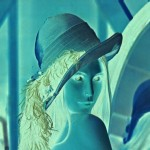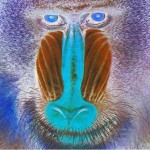# Image Processing Algorithms Part 7: Colour Inversion And Solarisation

## Colour inversion, also known as the negative effect, is one of the easiest effects to achieve in image processing. Colour inversion is achieved by subtracting each RGB colour value from the maximum possible value (usually 255).

In pseudo-code performing the colour inversion would go something like this:

```   colour = GetPixelColour(x, y)
invertedRed   = 255 - Red(colour)
invertedGreen = 255 - Green(colour)
invertedBlue  = 255 - Blue(colour)
PutPixelColour(x, y) = RGB(invertedRed, invertedGreen, invertedBlue)
```

To demonstrate the colour inversion effect we have here the ‘Lena’ and ‘mandrill’ images showing both the original and inverted versions:Original ‘Lena’ image (left) and inverted ‘Lena’ image (right)
(click images to enlarge)Original ‘mandrill’ image (left) and inverted ‘mandrill’ image (right)
(click images to enlarge)

Another effect which is related to colour inversion is the solarise effect. The difference between the solarise effect and colour inversion is that with the solarise effect only colour values above or below a set threshold are inverted.

In pseudo-code performing the solarise effect would go something like this:

```   colour = GetPixelColour(x, y)
If Red(colour) < threshold
solariseRed = 255 - Red(colour)
Else
solariseRed = Red(colour)
EndIf
If Green(colour) < threshold
solariseGreen = 255 - Green(colour)
Else
solariseGreen = Green(colour)
EndIf
If Blue(colour) < threshold
solariseBlue = 255 - Blue(colour)
Else
solariseBlue = Blue(colour)
EndIf
PutPixelColour(x, y) = RGB(solariseRed, solariseGreen, solariseBlue)
```

Alternatively you could change the ‘<‘ to a ‘>’ in the above code for a different solarise effect.

The images below show the inversion of colours below a threshold of 128 in the first instance and then the inversion of colours above a threshold of 128 in the second.

The method of solarisation described here is probably the most basic. There are more advanced methods of solarisation that use predefined or user-created curves to map the input colour values to the output colour values.